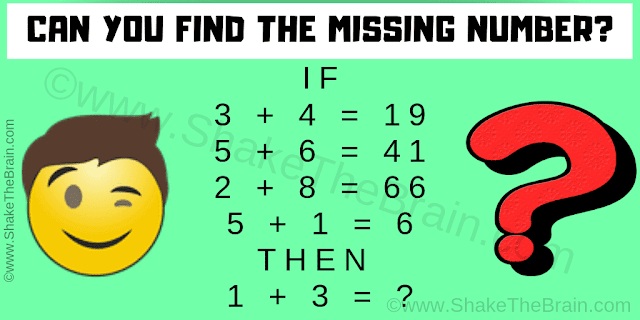This Math Tricky Riddle will challenge your brain. This math logic puzzle is dedicated to school going students. Solving this math logic puzzle will help you to test your logical reasoning and mathematical skills. Can you find the missing number by decode the logic used in these equations?Can you solve this Math Tricky Riddle?
Answer of this "Math Tricky Riddle", can be viewed by clicking on the answer button.